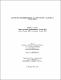## Quantum gravity effects in scalar, vector and tensor field propagation2016
##### Authors
Dutta, Anindita
University of Lethbridge. Faculty of Arts and Science
##### Publisher
Lethbridge, Alta : University of Lethbridge, Dept. of Physics and Astronomy
##### Abstract
Quantum theory of gravity deals with the physics of the gravitational field at Planck length scale (10^(−35) m). Even though it is experimentally hard to reach the Planck length scale, one can look for evidence of quantum gravity that is detectable in astrophysics. In this thesis, we try to find effects of loop quantum gravity corrections on observable phenomena. We show that the quantum fluctuation strain for LIGO data would be 10^(−125) on the Earth. The correction is, however, substantial near the black hole horizon. We discuss the effect of this for scalar field propagation followed by vector and tensor fields. For the scalar field, the correction introduces a new asymmetry; for the vector field, we found a new perturbation solution and for the tensor field, we found the corrected Einstein equations which are yet to solve. These will affect phenomena like Hawking radiation, black hole entropy and gravitational waves.
##### Keywords
black holes , canonical gravity , quantum gravity , scalar field , tensor field , vector field